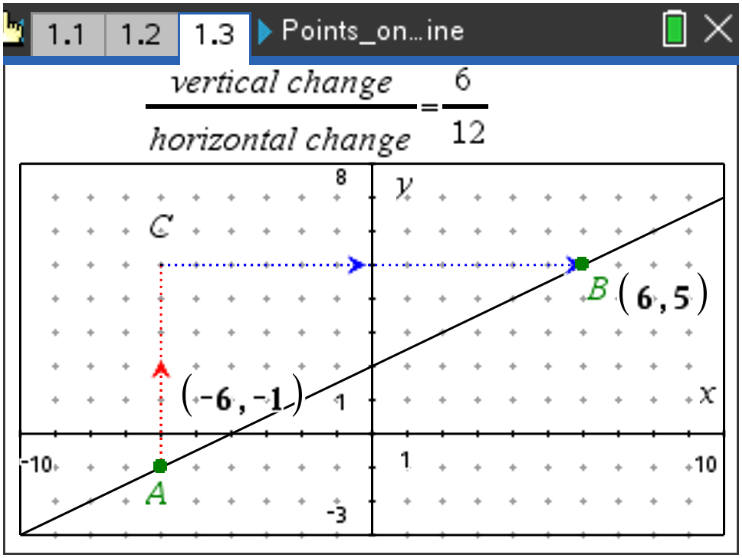### Mathematics lessons for IB® Diploma Programme

Analysis and Approaches | Functions

## Points, Lines, and Slopes (Oh My!)

### Objectives• Students will graph linear functions and determine properties such as slope and y-intercept, describe how both change as the graph is rotated and translated, and understand that the coordinates of a line are the solution set to the equation of the line.
• Students will show that lines with equal slopes are parallel and slopes that are negative reciprocals are perpendicular.
• Students will try to make a connection with how to understand these topics in IB Mathematics courses and on their final assessments.

### Vocabulary

• Parallel
• Perpendicular
• Reciprocal
• Slope